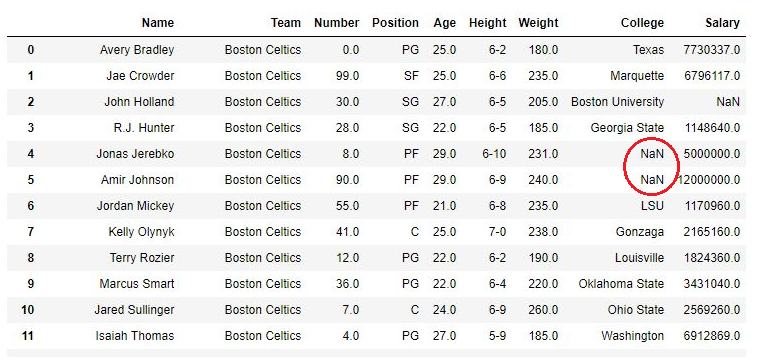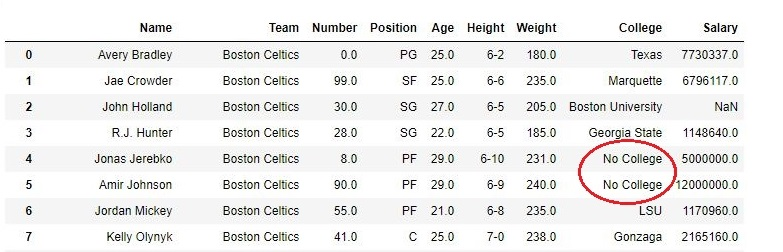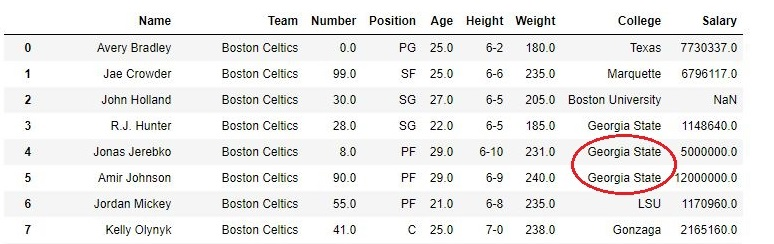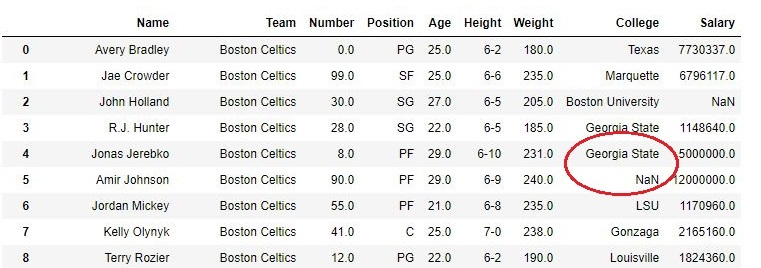# pandas dataframe 填充 NaN（填补缺失值）的方法 fillna 函数使用说明Pandas DataFrame 常用操作及基本知识点详解

## fillna 函数

DataFrame.fillna(value=None, method=None, axis=None, inplace=False, limit=None, downcast=None, **kwargs)

fillna 函数将用指定的值（value）或方式（method）填充 NA/NaN 等空值缺失值。

value

method

axis

inplace

limit

## 示例

### 通过常数填充 NaN

import pandas as pd

df = pd.read_csv("nba.csv")

DataFrame 对象 df 如下图：import pandas as pd

df["College"].fillna("No College", inplace = True)### 利用 method 参数填充 NaN

import pandas as pd

df["College"].fillna(method ='ffill', inplace = True)### 使用 limit 参数设置填充上限

import pandas as pd

df["College"].fillna(method ='ffill', limit = 1, inplace = True)